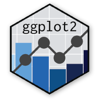Show Sidebar Hide Sidebar# geom_jitter in ggplot2

How to make a graph using geom_jitter.

### New to Plotly?

Plotly's R library is free and open source!
You can set up Plotly to work in online or offline mode.
We also have a quick-reference cheatsheet (new!) to help you get started!

### Version Check

Version 4 of Plotly's R package is now available!
Check out this post for more information on breaking changes and new features available in this version.

library(plotly)
packageVersion('plotly')

##  '4.9.1'


### Basic Jitter Plot

You can use the "text=" option to control what pops when you hover over each point. (Note: you might get an error message when running this function; ggplot does not recognize it but the plotly function does.)

library(plotly)
stringsAsFactors = FALSE)
district_density$cluster <- factor(district_density$cluster, levels=c("Pure urban", "Urban-suburban mix", "Dense suburban", "Sparse suburban", "Rural-suburban mix", "Pure rural"))
district_density$region <- factor(district_density$region, levels=c("West", "South", "Midwest", "Northeast"))

p <- ggplot(district_density,aes(x=cluster, y=dem_margin, colour=region)) +
geom_jitter(aes(text=paste("district: ", cd_code)), width=0.25, alpha=0.5, ) +
geom_hline(yintercept=0) +
theme(axis.text.x = element_text(angle = -30, hjust = 0.1)) +
labs(title = "Democratic performance in the 2018 House elections, by region and density",
x = "Density Index from CityLab",
y = "Democratic Margin of Victory/Defeat")
p <- ggplotly(p)

p


library(plotly)

p <- ggplot(district_density,aes(x=cluster, y=dem_margin)) +
geom_boxplot(fill=NA, alpha=0.5) +
geom_jitter(aes(colour=region, text=paste("district: ", cd_code)), width=0.25, alpha=0.5) +
geom_hline(yintercept=0) +
theme(axis.text.x = element_text(angle = -30, hjust = 0.1)) +
labs(title = "Democratic performance in the 2018 House elections, by region and density",
x = "Density Index from CityLab",
y = "Democratic Margin of Victory/Defeat")
p <- ggplotly(p)

p


### Facetting

library(plotly)

p <- ggplot(district_density,aes(x=region, y=dem_margin, colour=region)) +
geom_jitter(aes(text=paste("district: ", cd_code)), width=0.25, alpha=0.5, ) +
geom_hline(yintercept=0) +
facet_grid(.~cluster) +
theme(axis.text.x = element_text(angle = -30, hjust = 0.1)) +
labs(title = "Democratic performance in the 2018 House elections, by region and density",
x = "Density Index from CityLab",
y = "Democratic Margin of Victory/Defeat")
p <- ggplotly(p)

p


Tabulating the number of observations in each category, and adding these numbers to the graph.

library(plotly)
library(dplyr)

density_sum <- district_density %>%
group_by(cluster, region) %>%
dplyr::summarise(count = n())

p <- ggplot(district_density,aes(x=region, y=dem_margin, colour=region)) +
geom_jitter(aes(text=paste("district: ", cd_code)), width=0.25, alpha=0.5, ) +
geom_hline(yintercept=0) +
facet_grid(.~cluster) +
theme(axis.text.x = element_text(angle = -30, hjust = 0.1)) +
geom_text(data = density_sum, aes(label = count,
x = region, y = -90)) +
labs(title = "Democratic performance in the 2018 House elections, by region and density",
x = "Density Index from CityLab",
y = "Democratic Margin of Victory/Defeat")
p <- ggplotly(p)

p


### Customized Appearance

Centre the title, add colours to the facet label, rotate the labels, change the font, and remove the unnecessary legend.

library(plotly)

p <- ggplot(district_density,aes(x=region, y=dem_margin, colour=region)) +
geom_jitter(aes(text=paste("district: ", cd_code)), width=0.25, alpha=0.5, ) +
geom_hline(yintercept=0) +
facet_grid(.~cluster) +
geom_text(data = density_sum, aes(label = count,
x = region, y = -90)) +
theme(axis.text.x = element_text(angle = -30, hjust = 0.1),
plot.title = element_text(hjust = 0.5),
strip.background = element_rect(fill="lightblue")) +
labs(title = "Democratic performance in the 2018 House elections, by region and density",
x = "Density Index from CityLab",
y = "Democratic Margin of Victory/Defeat") +
theme(text = element_text(family = 'Fira Sans'),
legend.position = "none")
p <- ggplotly(p)

p


### Position Jitterdodge

Up to this point, we've subdivided points by making one category the x-axis, and facetting by the other. Another way is to make one category the x-axis, then use "position = dodge" so that the points are distinct rather than overlapping. Since we want points to be jittered and dodged, we can use geom_point with position_jitterdodge().

Make sure to specify the "group" variable: this graph specifies three potential grouping variables (cluster, region, cd_code), and position_jitterdodge can't tell which two to use unless specified. Further, you can use the ggplotly() function to specify what shows up on the tooltip.

library(plotly)

p <- ggplot(district_density,aes(x=cluster, y=dem_margin, colour=region,
district=cd_code, group=paste(cluster, region))) +
geom_point(position=position_jitterdodge(), alpha=0.5) +
geom_hline(yintercept=0) +
theme(axis.text.x = element_text(angle = -30, hjust = 0.1),
plot.title = element_text(hjust = 0.5)) +
labs(title = "Democratic performance in the 2018 House elections, by region and density",
x = "Density Index from CityLab",
y = "Democratic Margin of Victory/Defeat") +
theme(text = element_text(family = 'Fira Sans'))
p <- ggplotly(p, tooltip=c("district","y"))

p